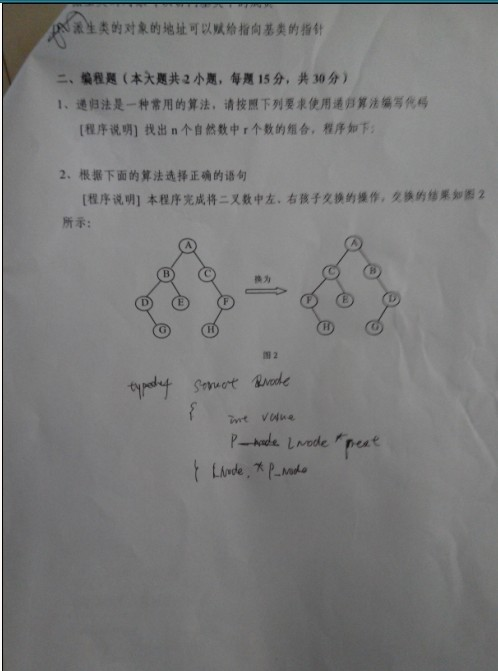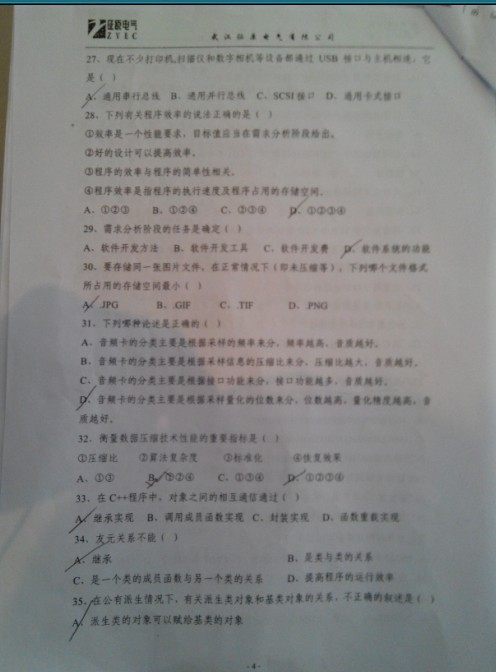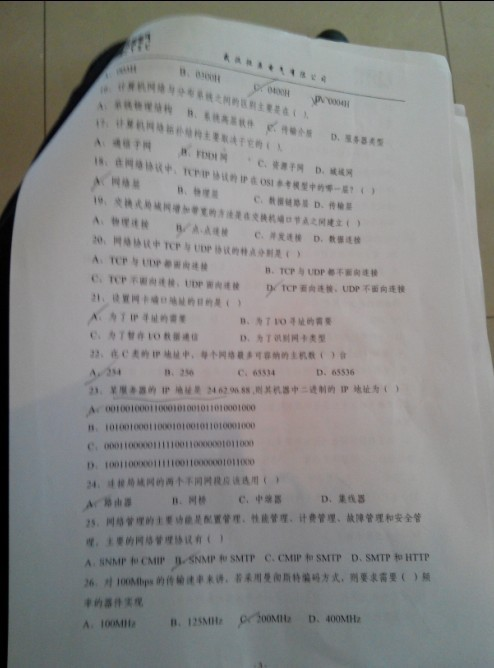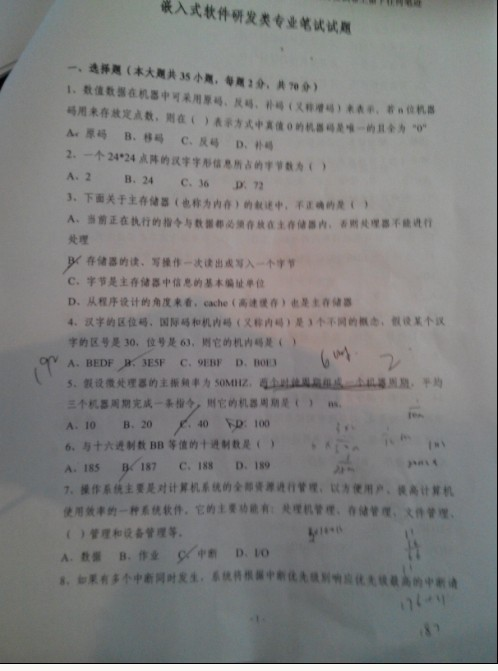100道c语言面试题

1、中兴、华为、慧通、英华达、微软亚洲技术中心等中

2、C 语言面试宝典(林锐《高质量编程第三版》)。

1、部分C 语言面试题中可能会参杂部分和C++ 相关的知

2、以上公司的面试题目已成为国内中小型企业公司出题

3、由于本人的能力有限加上时间仓促，本课件肯定存在

1、请填写bool , float,  指针变量 与“零值”比较的if

。例如int 变量n  与“零值”比较的if  语句为：
if ( n == 0 )
if ( n != 0 )

（1）请写出bool flag  与“零值”比较的if  语句：
【标准答案】if ( flag )    if ( !flag )
100 条经典 C语言笔试题目
（2）请写出float  x   与“零值”比较的if  语句：
【标准答案】 const float EPSINON = 0.00001;
if ((x >= - EPSINON) && (x <= EPSINON)

100 条经典 C语言笔试题目
（3）请写出char  *p   与“零值”比较的if  语句
【标准答案】 if (p == NULL)    if (p != NULL)

2、以下为Linux下的32 位C 程序，请计算sizeof 的

char  str[] = “Hello” ;
char   *p = str ;
i nt n = 10;

（1）sizeof (str ) =                  （2）s i zeof ( p ) =
（3）sizeof ( n ) =

【标准答案】

（1）6、（2）4 、（3 ）4

（4）void Func ( char str)
{
…… ;
}

（5）void * p = mall oc( 100 );

【标准答案】（4）4、（5）4

4、用变量a 给出下面的定义
e) 一个有10个指针的数组，该指针是指向一个整型数

f)  一个指向有10个整型数数组的指针;
g) 一个指向函数的指针，该函数有一个整型参数并返

h) 一个有10个指针的数组，该指针指向一个函数，该

【标准答案】e)int *  a;      f)int (*a)
g)int (*a)(int);   h) int (*a)(int)

5、设有以下说明和定义：
typedef u nion {long i; int k; char c;} DATE;
struct data { int cat; DATE cow; double dog;} too;
DATE max;

【标准答案】DATE是一个union, 变量公用空间.  里面最

20
data 是一个struct,  每个变量分开占用空间.  依次为int4 +
DATE20 + double8 = 32.

6、请问以下代码有什么问题：
int main()
{
char a;
char *str=&a;
strcpy(str,"hello");
printf(str);
return 0;
}
【标准答案】没有为str分配内存空间，将会发生异常

7、请问以下代码有什么问题：
char* s="AAA";
printf("%s",s);
s='B';
printf("%s",s);

【标准答案】"AAA" 是字符串常量。s是指针，指向这个

cosnt char* s="AAA";

8、int (*s)(int)  表示的是什么啊
【标准答案】int (*s)(int)  函数指针数组，每个指针

9、c和c++ 中的struct有什么不同？
【标准答案】c和c++ 中struct的主要区别是c中的struct

struct和class的主要区别在于默认的存取权限不同，
struct默认为public ，而class默认为private

10、void getmemory(char *p)
{
p=(char *) malloc(100);
strcpy(p,“hello world”);
}
int main( )
{
char *str=NULL;
getmemory(str);
printf(“%s/n”,str);
free(str);
return 0;
} 会出现什么问题？
【标准答案】程序崩溃，getmemory中的malloc 不能返回

11、char szstr;
strcpy(szstr,"0123456789");

【标准答案】长度不一样，出现段错误。
100 条经典 C语言笔试题目
12、数组和链表的区别？
【标准答案】数组：数据顺序存储，固定大小；

13、void main()
{
char aa;
printf(“%d”,strlen(aa));
}

【标准答案】sizeof()和初不初始化，没有关系，
strlen()和初始化有关，打印结果值未知。

14、给定结构struct A
{
char t:4;
char k:4;
unsigned short i:8;
unsigned long m;
}; 问sizeof(A) = ?
【标准答案】8

15、struct name1{
char str;
short x;
int num;
} ；求sizeof(name1)?
【标准答案】8

16、struct name2{
char str;
int num;
short x;
}; 求sizeof(name2)？
【标准答案】12

17、程序哪里有错误
wap( int* p1,int* p2 )
{
int * p;
*p = *p1;
*p1 = *p2;
*p2 = *p;
}

【标准答案】p 为野指针

19、(void *)ptr 和(*(void**))ptr 的结果是否相同？其

【标准答案】(void *)ptr 和(*(void**))ptr 值是相同的

20、要对绝对地址0x100000赋值，我们可以用
(unsigned int*)0x100000 = 1234;

，应该怎么做？
【标准答案】*((void (*)( ))0x100000 ) ( );

(void (*)())0x100000

*((void (*)())0x100000)();

22、关于内存的思考题（1）你能看出有什么问题？

23、关于内存的思考题（2）你能看出有什么问题？

25、关于内存的思考题（3）你能看出有什么问题？

26、关于内存的思考题（4）你能看出有什么问题？

27、关键字volatile有什么含意? 并给出三个不同的例

【参考答案】一个定义为volatile的变量是说这变量可

1). 并行设备的硬件寄存器（如：状态寄存器）
2). 一个中断服务子程序中会访问到的非自动变量
(Non-automatic variables)
3). 多线程应用中被几个任务共享的变量

28、嵌入式系统经常具有要求程序员去访问某特定的

【参考答案】这一问题测试你是否知道为了访问一绝对

。典型的类似代码如下：
int * ptr;
ptr=  (int *)0x67a9;
*ptr = 0xaa55;

29、头文件中的ifndef/define/endif 干什么用？
【标准答案】防止该头文件被重复引用。

30、#include  <filename.h>    和#include
“filename.h” 有什么区别？
【标准答案】对于#include  <filename.h>   ，编译器从

31、const   有什么用途？（请至少说明两种）
【标准答案】： （1）可以定义const   常量
（2）const  可以修饰函数的参数、返回值，甚至函数

32、static有什么用途？（请至少说明两种）
【标准答案】
1. 限制变量的作用域（static全局变量）；
2. 设置变量的存储域（static局部变量）。

33、堆栈溢出一般是由什么原因导致的？
【标准答案】没有回收垃圾资源。

34、如何引用一个已经定义过的全局变量？
【标准答案】可以用引用头文件的方式，也可以用
extern 关键字，如果用引用头文件方式来引用某个在

，那么在编译期间会报错，如果你用extern 方式引用

35、全局变量可不可以定义在可被多个.C 文件包含的

【标准答案】可以，在不同的C 文件中以static形式来声

36、队列和栈有什么区别？
【标准答案】队列先进先出，栈后进先出。

37、Heap与stack的差别。
【标准答案】Heap是堆，stack是栈。
Stack的空间由操作系统自动分配/释放，Heap上的空

Stack空间有限，Heap是很大的自由存储区
C 中的malloc 函数分配的内存空间即在堆上,C++中对

38、用宏定义写出swap（x，y），即交换两数。
【标准答案】
#define swap(x, y) (x)=(x)+(y);(y)=(x)–(y);(x)=(x)–(y);

39、写一个“标准”宏，这个宏输入两个参数并返回较

【标准答案】#define Min(X, Y) ((X)>(Y)?(Y):(X))// 结尾

40、带参宏与带参函数的区别(至少说出5点)？
【标准答案】

41、关键字volatile有什么含意？
【标准答案】提示编译器对象的值可能在编译器未监测

42、int main()
{
int x=3;
printf("%d",x);
return 1;
}

【标准答案】mian中，c标准认为0表示成功，非0表示

43、已知一个数组tabl e ，用一个宏定义，求出数据的

【标准答案】
#define NTBL(table) (sizeof(table)/sizeof(table))

44、A.c 和B.c两个c文件中使用了两个相同名字的
static变量,编译的时候会不会有问题?这两个static变量

【标准答案】static的全局变量，表明这个变量仅在本

extern 关键字。

45、static全局变量与普通的全局变量有什么区别？
【标准答案】 static全局变量只初使化一次，防止在其

46、static局部变量和普通局部变量有什么区别
【标准答案】static局部变量只被初始化一次，下一次

47、static函数与普通函数有什么区别？
【标准答案】static函数在内存中只有一份，普通函数在

。全局变量本身就是静态存储方式，静态全局变量当然也是静态存储方

static函数与普通函数作用域不同。仅在本文件。只在当前源文件中使

48、程序的局部变量存在于___ 中，全局变量存在于
____中，动态申请数据存在于___ 中。
【标准答案】程序的局部变量存在于栈(stack) 中，全局

heap）中。

49、什么是预编译，何时需要预编译：
【标准答案】１、总是使用不经常改动的大型代码体

２、程序由多个模块组成，所有模块都使用一组标准

50、用两个栈实现一个队列的功能？要求给出算法和

【参考答案】设2个栈为A,B, 一开始均为空.

(1)判断栈B 是否为空；
(2)如果不为空，则将栈A中所有元素依次pop 出并
push到栈B；
(3)将栈B 的栈顶元素pop 出；

51、对于一个频繁使用的短小函数,在C 语言中应用什

【标准答案】c用宏定义，c++ 用inline

52、1. 用预处理指令#define  声明一个常数，用以表

【参考答案】#define SECONDS_PER_YEAR (60 * 60
* 24 * 365)UL

53、Typedef 在C 语言中频繁用以声明一个已经存在的

#define dPS struct s *
typedef struct s * tPS;

【参考答案】这是一个非常微妙的问题，任何人答对这个问题（正当的原

dPS p 1,p2;
tPS p3,p4;

struct s * p1, p2;

54、在C++  程序中调用被C 编译器编译后的函数，

【标准答案】C++ 语言支持函数重载，C 语言不支持函

C++ 编译器则会产生像_foo_int_int之类的名字。 C++

55、请简述以下两个for  循环的优缺点。

【标准答案】

56、语句for(  ；1  ；) 有什么问题？它是什么意思？
【标准答案】死循环，和while(1)相同。

57、do……while和while……do有什么区别？
【标准答案】前一个循环一遍再判断，后一个判断以后

58、请写出下列代码的输出内容
#include <stdio.h>
int main()
{
int a,b,c,d;
a=10;
b=a++;
c=++a;
d=10*a++;
printf("b，c ，d：%d，%d，%d"，b，c，d ）;
return 0;
}
【标准答案】10，12，120

59、unsigned char *p1;
unsigned long *p2;
p1=(unsigned char *)0x801000;
p2=(unsigned long *)0x810000;

p2+5= ;
【标准答案】0x801005、0x810020

60、main()
{
int a={1,2,3,4,5};
int * ptr=(int*)(&a+1);
printf(“%d，%d”,*(a+1),*(ptr-1));
}

【标准答案】2,5

61、请问下面程序有什么错误?
int a,i,j,k;
for(k=0;k<=1000;k++)
for(j=0;j<250;j++)
for(i=0;i<60;i++)
a[i][j][k]=0;
【标准答案】把循环语句内外换一下。

62、以下是求一个数的平方的程序,请找出错误:
#define SQUARE(a)((a)*(a))
int a=5;
int b;
b=SQUARE(a++);
【标准答案】宏在预编译时会以替换的形式展开，仅仅

63、#define Max_CB 500
void LmiQueryCSmd(StructMSgCB * pmsg)
{
unsigned char ucCmdNum;
......
for(ucCmdNum=0;ucCmdNum<Max_CB;ucCmdN
um++)
{
......;
}
} 这段代码执行有什么问题？
【标准答案】死循环
unsigned char //无符号字符型表示范围0~255
char // 有符号字符型 表示范围-128~127

64、嵌入式系统中经常要用到无限循环，你怎么用C

【标准答案】while(1){}或者for(;;)
100 条经典 C语言笔试题目
65、程序输出结果是？
【标准答案】8 ，10，12，14，16

66、
【标准答案】16

67、int modifyvalue()

return(x+=10);
}
int c hangevalue(int x )
{
return(x+=1);
}
void m ain()
{
int x =10;
x++;
changevalue(x);
x++;
modifyvalue();
printf("First output:%dn",x);
x++;
changevalue(x);
printf("Second output:%dn",x);
modifyvalue();
printf("Thirdoutput:%dn",x);
}输出?
【标准答案】12、13、13

68、不能做switch()的参数类型是：
【标准答案】switch 的参数不能为实型。

69、请写出下列代码的输出内容
＃i nclude<stdio.h>
main()
{
int a,b,c,d;
a=10;
b=a++;
c=++a;
d=10*a++;
printf("b，c ，d：%d，%d，%d"，b，c，d ）;
return 0;
}
【标准答案】10，12，120

70、找出程序的错误。
【标准答案】

71、一语句实现x是否为2 的若干次幂的判断。
【参考答案】 void mai n()
{
i nt a;
scanf(“%d”,&a);
printf(“%c”,(a)&(a-1)?’n’:’y’); //   若是打印y，否则n
}

72、中断是嵌入式系统中重要的组成部分，这导致了很多编译开发商提

__interrupt 。下面的代码就使用了__interrupt 关键字去定义了一个中断服

{
printf(" Area = %f", area);
return area;
}
【参考答案】这个函数有太多的错误了，以至让人不知从何说起了：
1). ISR 不能返回一个值。如果你不懂这个，那么你不会被雇用的。
2). ISR 不能传递参数。如果你没有看到这一点，你被雇用的机会等同第

3). 在许多的处理器/编译器中，浮点一般都是不可重入的。有些处理器/编

4). 与第三点一脉相承，printf() 经常有重入和性能上的问题。如果你丢掉

73、下面的代码输出是什么，为什么？
void foo(void)
{
unsigned int a = 6;
int b = -20;
(a+b> 6)? puts("> 6") : puts("<= 6");
}
【参考答案】这个问题测试你是否懂得C 语言中的整数自动转换原则，

74、评价下面的代码片断：
unsigned int zero = 0;
unsigned int compzero =  0xFFFF;
/*1‘s complement of zero */
【参考答案】对于一个int型不是16位的处理器为说，上面的代码是不正

unsigned int compzero = ~0;

PC机程序往往把硬件作为一个无法避免的烦恼。

75、下面的代码片段的输出是什么，为什么？
char *ptr;
if ((ptr = (char *)malloc(0)) == NULL)
puts("Gota null pointer");
else
puts("Gota valid pointer");
【参考答案】这个你可以先大胆猜测下，然后再用你的

100 条经典 C语言笔试题目

76、编写strcpy 函数

const char *strSrc);其中strDest是目的字符串，
strSrc 是源字符串。
（1）不调用C++/C 的字符串库函数，请编写函数
strcpy 。
（2）strcpy 能把 strSrc 的内容复制到strDest，为什

77、写出二分查找的代码。
【参考答案】 int b inary_search(int* arr, int k ey, int n)
{
int low =  0;
int h igh = n - 1 ;
int m id;
while (low <= high)
{
mid = (high + low) / 2;
if (arr[mid] > k)
high = mid -1 ;
else if (arr[mid] < k)
low = mid + 1;
else
return mid;
}
return -1;
}

78、请编写一个C 函数，该函数给出一个字节中被置
1  的位的个数。
【参考答案】
unsigned int T estAsOne0(char log)

int i;
unsigned int num=0, val;
for(i=0; i<8; i++)

va l =  log >>  i;  // 移位
val & = 0x01;  // 与1 相与
if(val)
num++;

return num;

79、请编写一个C 函数，该函数将给定的一个字符串

【参考答案】
int Invert(char* str)

int num =0;
while(*str!='\0')

int d igital=*str-48;
num=num*10+digital;
str=str+1;

return num;

80、请编写一个C 函数，该函数将给定的一个整数转

【参考答案】
void IntToCharChange(int num,  char* pval)

char strval;
int i , j;
int val0 = 0;
int val1 = 0;
val0 = num;
for(i=0; i<100; i++)

val1 = val0 % 10; //取余
val0 = val0 / 10; // 取整
strval[i] = val1 + 48;  // 数字—字符
if(val0 < 10)

i++;
strval[i] = val0 + 48;
break;

for(j=0; j<=i; j++)  // 倒置
pval[j] = strval[i-j];
pval[j] = '\0';
}

81、实现strcmp 函数。
【参考答案】
int m ystrcmp(const c har* str1, const char* str2)
{
assert((str1 != NULL) && (str2 != NULL));
int r et = 0;
while (!(ret = *(unsigned char*)str1 - * (unsigned char*)str2) && *str2)
{
str1++;
str2++;
}
if (ret > 0)
ret = 1;
else if (ret < 0)
ret = -1;
return ret;
}

82、请编写一个C 函数，该函数将一个字符串逆序。
【参考答案】
void AntitoneValue(cha r* father, char* child)

int i ;
char source;
int j = 0;
while(father[j]) //放入source ，[j] 为长度

source[j] = father[j];
j++;
if(j > 99)
return;

source[j] = '\0';
for(i=0; i<j; i++)
child[i] = source[j-i-1];  // 反序
child[i] = '\0';

83、请编写一个C 函数，该函数在给定的内存区域搜

【参考答案】
int s earch(char* cpSource, intn , char ch)  // 起始地址，搜索长度，目标字符
{
int i;
for(i=0; i<n && *(cpSource+i) != ch; ++i);
return i;
}

84、请编写一个C 函数，该函数在一个字符串中找到

【参考答案】
int C hildString(char*p)     // 自己写
{
char *q =p;
int s tringlen=0, i=0,j=1,len=0,maxlen=1;
while(*q!=’\0’)          //不能用strlen, 求得长度stringlen
{
Stringlen++;
q++;
}
while( i<  String len )
{
if(*(p+i)==*(p+j)& & j< St ri ngle n )
{
len++;                    // 统计子串长度
i++;
j++;
}
else
{
if(len>maxlen)           // 统计最大子串长度
{
maxlen=len+1;
len=0;
}
else
len=0;
i++;
j++;
}
}
retu rn   ma xlen;
}

85、华为面试题：怎么判断链表中是否有环？
【参考答案】答：用两个指针来遍历这个单向链表，第

{
while( t1->next && t2->next)
{
t1 = t1->next;
if (NULL == (t2 = t2->next->next))
return 0; // 无环
if (t1 == t2)
return 1;
}
return 0;
}

86、有一浮点型数组A, 用C 语言写一函数实现对浮点

【参考答案】
void BubbleSort(double a rr[], int n)

int i，j ；
int e xchange = 1 ；// 交换标志
for(i=1;i<n;i++)
{ // 最多做n-1趟排序
exchange=0 ；// 本趟排序开始前，交换标志应为假
for(j=n-1;j>=i ；j--) //对当前无序区R[i..n]自下向上扫描
if(arr[j+1] > arr [ j])
{// 交换记录
arr=arr[j+1]；//R不是哨兵，仅做暂存单元
arr[j+1]=arr[j]；
arr[j]=arr；
exchange=1 ；// 发生了交换，故将交换标志置为真

if(!exchange) // 本趟排序未发生交换，提前终止算法
return ；
} //endfor(外循环)
}

87、实现双向链表删除一个节点P，在节点P 后插入一

【参考答案】
// 删除操作
{
if(!(p=G e tElemP_DuL(L,i))) return ERROR;
e=p->data;
p->prior->next=p->next;
p->next->prior=p->pror;
free(p);
return OK;
}
// 插入操作
{
if(!(p=G e tElemP_DuL(L,i)))
return ERROR;
return ERROR;
s->data=e;
s->pri or =p;
p-> next -> prior =s;
p->next=s;
s->next=p->next->next;
return OK;
}

88、把一个链表反向。
【参考答案】从第一个元素开始，ps指向他，将他（

{
while(ps)

pe->next = ps->next;
ps =  pe->next;
}
}

89、将二维数组行列元素互换，存到另一个数组中。
【参考答案】
#include <stdio.h>
main()
{   int a ={{1,2,3},{4,5,6}};
int b,i,j;
printf("array a :\n");
for(i=0;i<=1;i++)
{   for(j=0;j<=2;j++)
{   printf("%5d",a[i][j]);
b[j][i]=a[i][j];
}
printf("\n");
}
printf("array b :\n");
for(i=0;i<=2;i++)
{   for(j=0;j<=1;j++)
printf("%5d",b[i][j]);
printf("\n");
}
}

90、输入一行字符，统计其中有多少个单词。
【参考答案】
#include <stdio.h>
main()
{
char str i ng;
int i,num=0,word=0;
char c;
gets(string);
for(i=0;(c=string[i])!='\0';i++)
if(c==' ')
word=0;
else if(word==0)
{
word=1;  num++;   }
printf("There are %d word s in the line\n",num);
}

91、写一个内存拷贝函数,不用任何库函数.就是前些时

【参考答案】
void* m e mcpy(void* pvTo, const void* pvFrom, size_t size)
{
assert((pvTo != NULL) && (pvFrom ! = NULL));
byte* pbTo= pvTo;
byte* pbFrom = pbFrom;
while (size-- >  0)
{
*pbTo++ = *pbFrom++;
}
return pvTo;
}

92、有1、2、3 、4个数字，能组成多少个互不相同且

【参考答案】
#inc lude  "stdio.h"
#include "conio.h"
main()
{
int i,j,k;
printf("\n");
for(i=1;i<5;i++) /* 以下为三重循环*/
for(j=1;j<5;j++)
for (k=1;k<5;k++)
{
if (i!=k&&i!=j&&j!=k) /* 确保i、j、k三位互不相同*/
pr intf("%d,%d,%d\n",i,j,k);
}
getch();
}

93、取一个整数a从右端开始的4～7位。
【参考答案】
main()
{
unsigned a,b,c,d;
scanf("%o",&a);
b=a>>4;
c=~(~0<<4);
d=b&c;
printf("%o\n%o\n",a,d);

}

94、打印出杨辉三角形（要求打印出10行如下图）。
【参考答案】
main()
{
int i,j;
int a ;
printf("\n");
for(i=0;i<10;i++)
{
a[i]=1;
a[i][i]=1;
}
for(i=2;i<10;i++)
for(j=1;j<i;j++)
a[i][j]=a[i-1][j-1]+a[i-1][j];
for(i=0;i<10;i++)
{
for(j=0;j<=i;j++)
printf("%5d",a[i][j]);
printf("\n");
}
getch();
}

95、实现strcmp 函数。
100 条经典 C语言笔试题目
96、写一个函数，求一个字符串的长度，在main函数

【参考答案】
main()
{
intlen;
char *s tr;
scanf("%s",str);
len=length(str);
printf("the s tring has %d characters.",len);
getch();
}
length(p)
char *p;
{
int n ;
n=0;
while(*p!='\0')
{
n++;
p++;
}
return n;
}

97、809*??=800*??+9*??+1  其中??代表的两位数
,8*?? 的结果为两位数，9*??的结果为3位数。求??代

【参考答案】output(long b,long i)
{
printf("\n%ld/%ld=809*%ld+%ld",b,i,i,b%i);
}
main()
{
long int a ,b,i;
a=809;
for(i=10;i<100;i++)
{
b=i*a+1;
if(b>=1000&&b<=10000&&8*i<100&&9*i>=100)
output(b,i);
}
getch();
}

98、某个公司采用公用电话传递数据，数据是四位的整数，在

【参考答案】
main()
{
int a ,i,aa,t;
scanf( " % d " ,&a) ;
aa=a %10;
aa=a %100/10;
aa=a %1000/100;
aa=a /1000;
for(i=0 ; i<=3 ;i++)
{
aa [i]+=5;
aa[i] %=10;
}
for(i=0;i<=3/2;i++)
{
t=aa[i];
aa[i]= aa[3- i];
aa[3- i]= t ;
}
for(i=3;i>=0;i--)
prin tf("% d ", a [i]);
getch();
}

99、计算字符串中子串出现的次数。
【参考答案】
main()
{
char str1,str2,*p1,*p2;
int sum=0;
scanf("%s%s",str1,str2);
p1=str1;p2=str2;
while(*p1!='\0')
{
if(*p1==*p2)
{
while(*p1==*p2&&*p2!='\0')
{
p1++;
p2++;
}
}
else
p1++;
if(*p2=='\0')
sum++;
p2=str2;
}
printf("%d",sum);
getch();

100 、有两个磁盘文件A和B, 各存放一行字母，要求把

【参考答案】
100 条经典 C语言笔试题目
main()
{
FILE *fp;
in t i,j,n,ni;
char c,t,ch;
if((fp=fopen("A","r "))==NULL)
{
printf("file A cannot  be opened\n");exit(0);
}
printf("\nA contents are :\n");
for(i=0;(ch=fgetc(fp))!=EOF ; i++)
{
c[i]=ch;pu tchar(c[i]);
}
fclose(fp);
ni=i;
if((fp=fopen("B","r "))==NULL)
{
printf("file B cannot  be opened\n");exit(0);
}
printf("\nB contents are :\n");
for(i=0;(ch=fgetc(fp))!=EOF ; i++)
{
c[i]=ch;pu tchar(c[i]);
}
fclose(fp);
n=i;
for(i=0;i<n;i++)
for(j=i+1;j<n;j++)
if(c[i]>c[j]){t=c[i ];c[i]=c[j];c[j]=t;}
printf("\nC file is:\n");
fp =f open("C","w");
for(i=0;i<n;i++){putc(c[i ],fp);putchar(c[i]);}
fclose(fp);
getch();
}